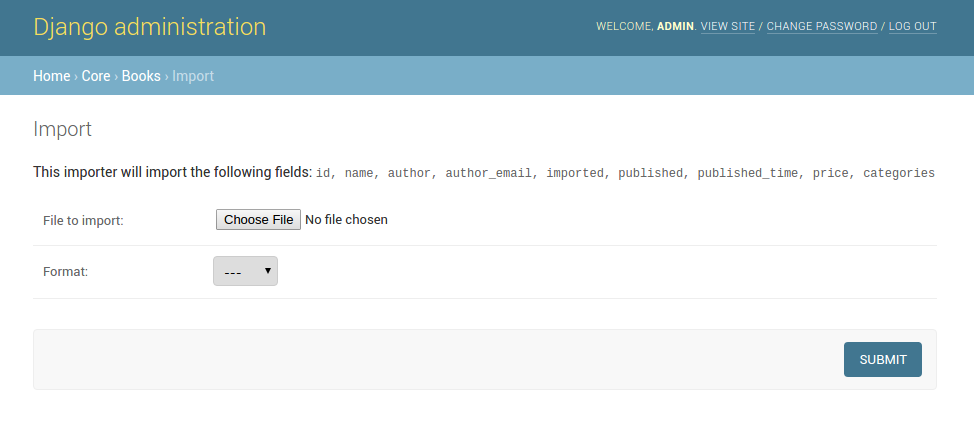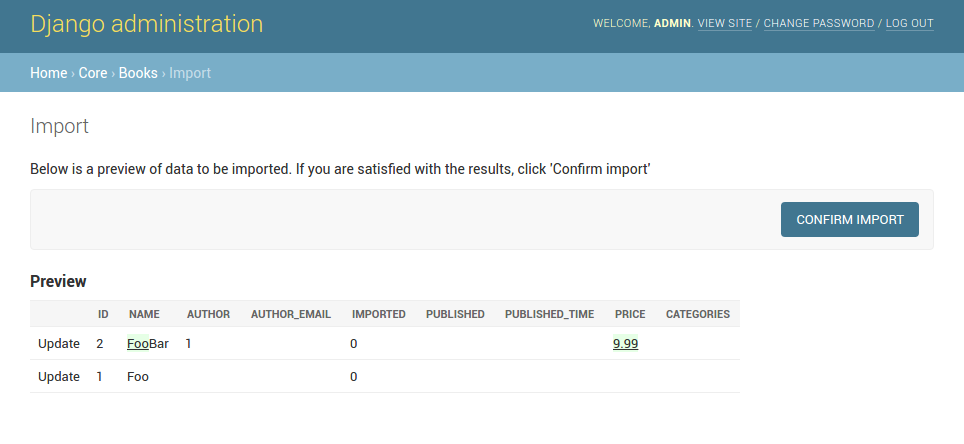# Getting started¶

For example purposes, we’ll use a simplified book app. Here is our `models.py`:

```# app/models.py

class Author(models.Model):
name = models.CharField(max_length=100)

def __str__(self):
return self.name

class Category(models.Model):
name = models.CharField(max_length=100)

def __str__(self):
return self.name

class Book(models.Model):
name = models.CharField('Book name', max_length=100)
author = models.ForeignKey(Author, blank=True, null=True)
author_email = models.EmailField('Author email', max_length=75, blank=True)
imported = models.BooleanField(default=False)
published = models.DateField('Published', blank=True, null=True)
price = models.DecimalField(max_digits=10, decimal_places=2, null=True, blank=True)
categories = models.ManyToManyField(Category, blank=True)

def __str__(self):
return self.name
```

## Creating import-export resource¶

To integrate django-import-export with our `Book` model, we will create a `ModelResource` class in `admin.py` that will describe how this resource can be imported or exported:

```# app/admin.py

from import_export import resources
from core.models import Book

class BookResource(resources.ModelResource):

class Meta:
model = Book
```

## Exporting data¶

Now that we have defined a `ModelResource` class, we can export books:

```>>> from app.admin import BookResource
>>> dataset = BookResource().export()
>>> print(dataset.csv)
id,name,author,author_email,imported,published,price,categories
2,Some book,1,,0,2012-12-05,8.85,1
```

## Customize resource options¶

By default `ModelResource` introspects model fields and creates `Field`-attributes with an appropriate `Widget` for each field.

To affect which model fields will be included in an import-export resource, use the `fields` option to whitelist fields:

```class BookResource(resources.ModelResource):

class Meta:
model = Book
fields = ('id', 'name', 'price',)
```

Or the `exclude` option to blacklist fields:

```class BookResource(resources.ModelResource):

class Meta:
model = Book
exclude = ('imported', )
```

An explicit order for exporting fields can be set using the `export_order` option:

```class BookResource(resources.ModelResource):

class Meta:
model = Book
fields = ('id', 'name', 'author', 'price',)
export_order = ('id', 'price', 'author', 'name')
```

The default field for object identification is `id`, you can optionally set which fields are used as the `id` when importing:

```class BookResource(resources.ModelResource):

class Meta:
model = Book
import_id_fields = ('isbn',)
fields = ('isbn', 'name', 'author', 'price',)
```

When defining `ModelResource` fields it is possible to follow model relationships:

```class BookResource(resources.ModelResource):

class Meta:
model = Book
fields = ('author__name',)
```

Note

Following relationship fields sets `field` as readonly, meaning this field will be skipped when importing data.

By default all records will be imported, even if no changes are detected. This can be changed setting the `skip_unchanged` option. Also, the `report_skipped` option controls whether skipped records appear in the import `Result` object, and if using the admin whether skipped records will show in the import preview page:

```class BookResource(resources.ModelResource):

class Meta:
model = Book
skip_unchanged = True
report_skipped = False
fields = ('id', 'name', 'price',)
```

Resources

## Declaring fields¶

It is possible to override a resource field to change some of its options:

```from import_export.fields import Field

class BookResource(resources.ModelResource):
published = Field(attribute='published', column_name='published_date')

class Meta:
model = Book
```

Other fields that don’t exist in the target model may be added:

```from import_export.fields import Field

class BookResource(resources.ModelResource):
myfield = Field(column_name='myfield')

class Meta:
model = Book
```

Fields
Available field types and options.

## Advanced data manipulation on export¶

Not all data can be easily extracted from an object/model attribute. In order to turn complicated data model into a (generally simpler) processed data structure on export, `dehydrate_<fieldname>` method should be defined:

```from import_export.fields import Field

class BookResource(resources.ModelResource):
full_title = Field()

class Meta:
model = Book

def dehydrate_full_title(self, book):
return '%s by %s' % (book.name, book.author.name)
```

In this case, the export looks like this:

```>>> from app.admin import BookResource
>>> dataset = BookResource().export()
>>> print(dataset.csv)
full_title,id,name,author,author_email,imported,published,price,categories
Some book by 1,2,Some book,1,,0,2012-12-05,8.85,1
```

## Customize widgets¶

A `ModelResource` creates a field with a default widget for a given field type. If the widget should be initialized with different arguments, set the `widgets` dict.

In this example widget, the `published` field is overridden to use a different date format. This format will be used both for importing and exporting resource.

```class BookResource(resources.ModelResource):

class Meta:
model = Book
widgets = {
'published': {'format': '%d.%m.%Y'},
}
```

Widgets
available widget types and options.

## Importing data¶

Let’s import some data!

 ```1 2 3 4 5 6 7 8 9``` ```>>> import tablib >>> from import_export import resources >>> from core.models import Book >>> book_resource = resources.modelresource_factory(model=Book)() >>> dataset = tablib.Dataset(['', 'New book'], headers=['id', 'name']) >>> result = book_resource.import_data(dataset, dry_run=True) >>> print(result.has_errors()) False >>> result = book_resource.import_data(dataset, dry_run=False) ```

In the fourth line we use `modelresource_factory()` to create a default `ModelResource`. The ModelResource class created this way is equal to the one shown in the example in section Creating import-export resource.

In fifth line a `Dataset` with columns `id` and `name`, and one book entry, are created. A field for a primary key field (in this case, `id`) always needs to be present.

In the rest of the code we first pretend to import data using `import_data()` and `dry_run` set, then check for any errors and actually import data this time.

Import data workflow
for a detailed description of the import workflow and its customization options.

### Deleting data¶

To delete objects during import, implement the `for_delete()` method on your `Resource` class.

The following is an example resource which expects a `delete` field in the dataset. An import using this resource will delete model instances for rows that have their column `delete` set to `1`:

```class BookResource(resources.ModelResource):
delete = fields.Field(widget=widgets.BooleanWidget())

def for_delete(self, row, instance):
return self.fields['delete'].clean(row)

class Meta:
model = Book
```

## Signals¶

To hook in the import export workflow, you can connect to `post_import`, `post_export` signals:

```from django.dispatch import receiver
from import_export.signals import post_import, post_export

def _post_import(model, **kwargs):
# model is the actual model instance which after import
pass

def _post_export(model, **kwargs):
# model is the actual model instance which after export
pass
```

### Exporting¶

#### Exporting via list filters¶

Admin integration is achieved by subclassing `ImportExportModelAdmin` or one of the available mixins (`ImportMixin`, `ExportMixin`, `ImportExportMixin`):

```# app/admin.py
from .models import Book

resource_class = BookResource

```A screenshot of the change view with Import and Export buttons.A screenshot of the import view.A screenshot of the confirm import view.

Another approach to exporting data is by subclassing `ImportExportActionModelAdmin` which implements export as an admin action. As a result it’s possible to export a list of objects selected on the change list page:

```# app/admin.py

pass
```A screenshot of the change view with Import and Export as an admin action.

Note that to use the `ExportMixin` or `ExportActionMixin`, you must declare this mixin before `admin.ModelAdmin`:

```# app/admin.py

pass
```

Note that `ExportActionMixin` is declared first in the example above!

### Importing¶

It is also possible to enable data import via standard Django admin interface. To do this subclass `ImportExportModelAdmin` or use one of the available mixins, i.e. `ImportMixin`, or `ImportExportMixin`. Customizations are, of course, possible.

It is possible to modify default import forms used in the model admin. For example, to add an additional field in the import form, subclass and extend the `ImportForm` (note that you may want to also consider `ConfirmImportForm` as importing is a two-step process).

To use the customized form(s), overload `ImportMixin` respective methods, i.e. `get_import_form()`, and also `get_confirm_import_form()` if need be.

For example, imagine you want to import books for a specific author. You can extend the import forms to include `author` field to select the author from.

Customize forms:

```from django import forms

class CustomImportForm(ImportForm):
author = forms.ModelChoiceField(
queryset=Author.objects.all(),
required=True)

class CustomConfirmImportForm(ConfirmImportForm):
author = forms.ModelChoiceField(
queryset=Author.objects.all(),
required=True)
```

Customize `ModelAdmin`:

```class CustomBookAdmin(ImportMixin, admin.ModelAdmin):
resource_class = BookResource

def get_import_form(self):
return CustomImportForm

def get_confirm_import_form(self):
return CustomConfirmImportForm

def get_form_kwargs(self, form, *args, **kwargs):
# pass on `author` to the kwargs for the custom confirm form
if isinstance(form, CustomImportForm):
if form.is_valid():
author = form.cleaned_data['author']
kwargs.update({'author': author.id})
return kwargs

To further customize admin imports, consider modifying the following `ImportMixin` methods: `get_form_kwargs()`, `get_import_resource_kwargs()`, `get_import_data_kwargs()`.